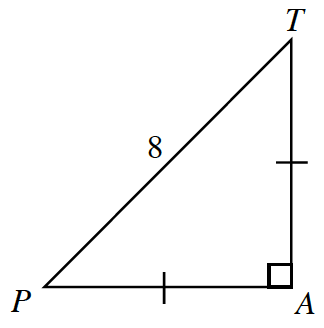### Home > PC3 > Chapter 1 > Lesson 1.1.5 > Problem1-49

1-49.

$ΔPAT$ is an isosceles right triangle with $PA=TA$1. Calculate the exact length of $\overline {\textit{PA}}$. Express your answer in simple radical form.

Use the Pythagorean Theorem. Since both legs are the same length, $TA=PA$. Label these sides $x$.

2. What is the exact value of $\sin(P)$?

Use the value found in part (a) in the formula:

$\operatorname { sin }(P) = \frac{\text{opposite}}{\text{hypotenuse}}$

3. What is the exact value of $\cos(P)$?

$\operatorname { cos }(P) = \frac{\text{adjacent}}{\text{hypotenuse}}$